VARIN ANAND — Published On January 31, 2022 and Last Modified On March 15th, 2022

2. Importance of Non-Linearity/Activation Functions
3. Activation Functions (Sigmoid, Tanh, ReLU, Leaky ReLU, ELU, Softmax) and their implementation
5. Endnotes

The work of the gradient descent algorithm is to update the weights in such a way that the loss function is minimum.

The updation rule is: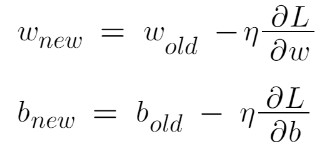Image Source: Author

We basically have to move in the direction opposite to the gradient. This concept is derived from the Taylor series.

Now, let’s understand what these derivatives are and how to calculate them: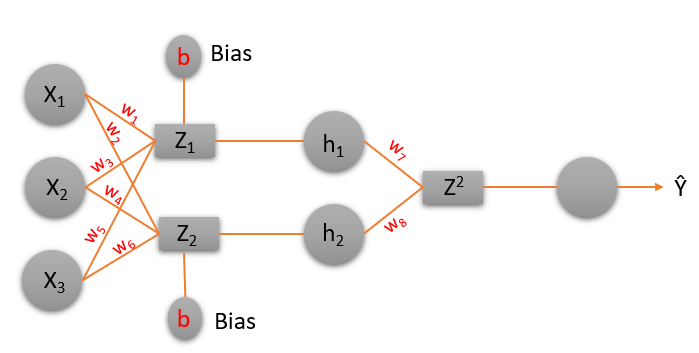Image Source: Author

Now from the image above, we can calculate the values of Z1, Z2, etc, and all the derivates like in the images below.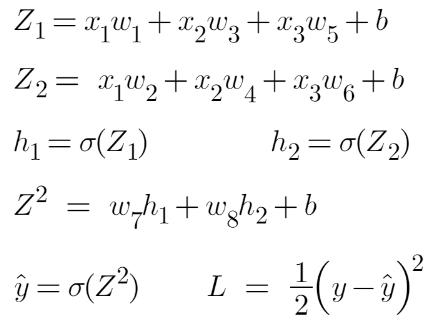Image Source: Author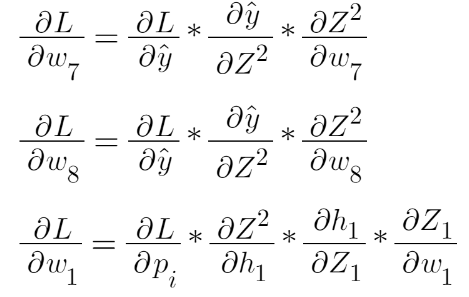Image Source: Author

We use the chain rule to find these derivatives and then put them in the gradient descent equation to update all the weights and biases.

## Importance of Non-Linearity/Activation Functions:

Activation functions are used to introduce non-linearity. What exactly is non-linearity and why is it important to introduce non-linearity? Let’s understand it with an example.

Non-linearity in a neural network simply means a function that is able to classify the class of a function that is divided by a decision boundary that is not linear.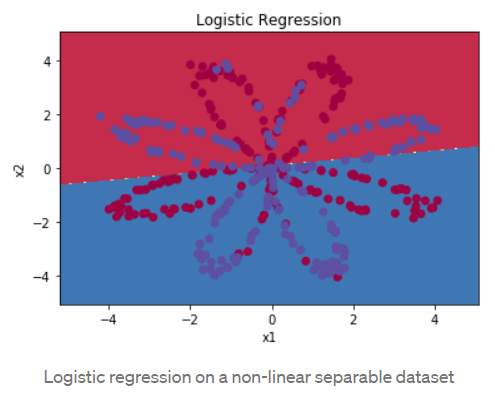Image Source – https://medium.com/ml-cheat-sheet/understanding-non-linear-activation-functions-in-neural-networks-152f5e101eeb

It is very hard to find any example in the real world which follows this linearity and hence we need some functions that can approximate a non-linear phenomenon.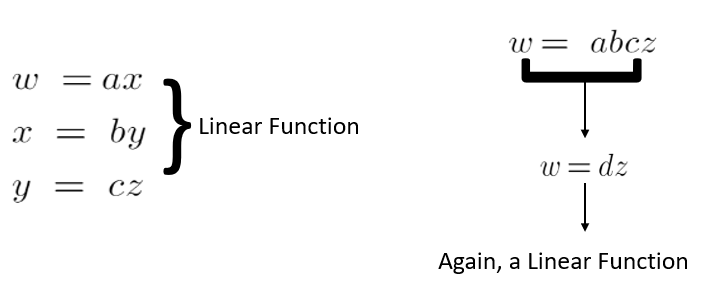If we take all linear functions then the output is nothing but a constant multiplication by the input.

Similarly in a Neural Network, if we do not take non-linearity into account, then the output is just a summation of inputs and weights. No matter how many layers it had, our Neural Network would behave just like a single-layer perceptron, because summing these layers would give you just another linear function (see diagram above).

## Activation Functions

(i) Step Activation Function: The Step activation function is used in the perceptron network. This is usually used in single-layer networks to convert to an output that is binary (0 or 1) or Bipolar (-1 or 1). These are called Binary Step Function and Bipolar Step Function Respectively. Here if the input value to a function is greater than a threshold value then the output is 1 (neuron will fire) otherwise 0 or -1 in the case of Bipolar Step Function (neuron will not fire).

(ii) Sigmoid Functions – The step activation function which is a logic used in perceptron is that if the value is greater than the threshold value then the output is 1 (neuron will fire) otherwise 0 (neuron will not fire) and this logic is used by perceptron is very harsh.

For eg: Suppose you want to watch a movie and decide whether you like it or not. Consider that we have only 1 input (x1-critics rating which lies between 0 and 1). Now if the threshold is 0.5 and the critics rating = 0.51 then we would like the movie and if the critic rating =0.49 then we would dislike the movie. This is what harsh means here.

WHY SIGMOID?

Sigmoid functions are broadly used in Back Propagation Networks because of the relationship between the value of the function and the value of the derivative at that point. This reduces overall computation overload.

There are two types of sigmoidal functions:

1. Binary Sigmoid
2. Bipolar Sigmoid

Binary Sigmoid Function:

This is also known as logistic sigmoid function. Its range lies between 0 and 1. The Sigmoid function gives the output in probability and it is smoother than the perceptron function. If w(t)x tends to infinity then the output gets close to If w(t)x tends to negative infinity the output gets close to 0.

Graph: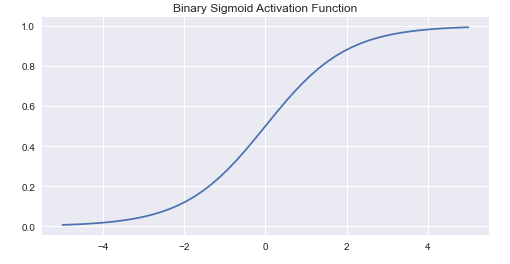Image Source: Author

Code Snippet:

```import numpy as np
import matplotlib.pyplot as plt
import numpy as np
plt.style.use('seaborn')
plt.figure(figsize=(8,4))
def SigmoidBinary(t):
return 1/(1+np.exp(-t))
t = np.linspace(-5, 5)
plt.plot(t, SigmoidBinary(t))
plt.title('Binary Sigmoid Activation Function')
plt.show()```

Bipolar Sigmoid Function: This is the function from where the Hyperbolic Tan Function was derived from. Here (lambda) represents the steepness factor. The range of this function is between -1 and 1. For the hyperbolic tangent function, the value of the steepness factor is 2.

Graph: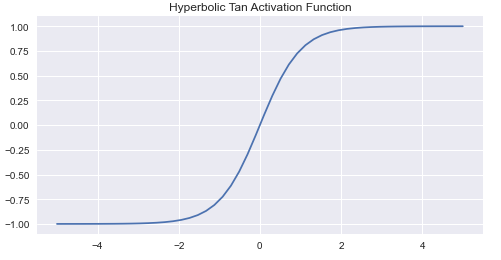Image Source: Author

Code Snippet:

```import numpy as np
import matplotlib.pyplot as plt
import numpy as np
plt.style.use('seaborn')
plt.figure(figsize=(8,4))
def HyperbolicTan(t):
return np.tanh(t)
t = np.linspace(-5, 5)
plt.plot(t, HyperbolicTan(t))
plt.title('Hyperbolic Tan Activation Function')
plt.show()```

The following image shows the relationship between the Binary and Bipolar Functions and their derivatives: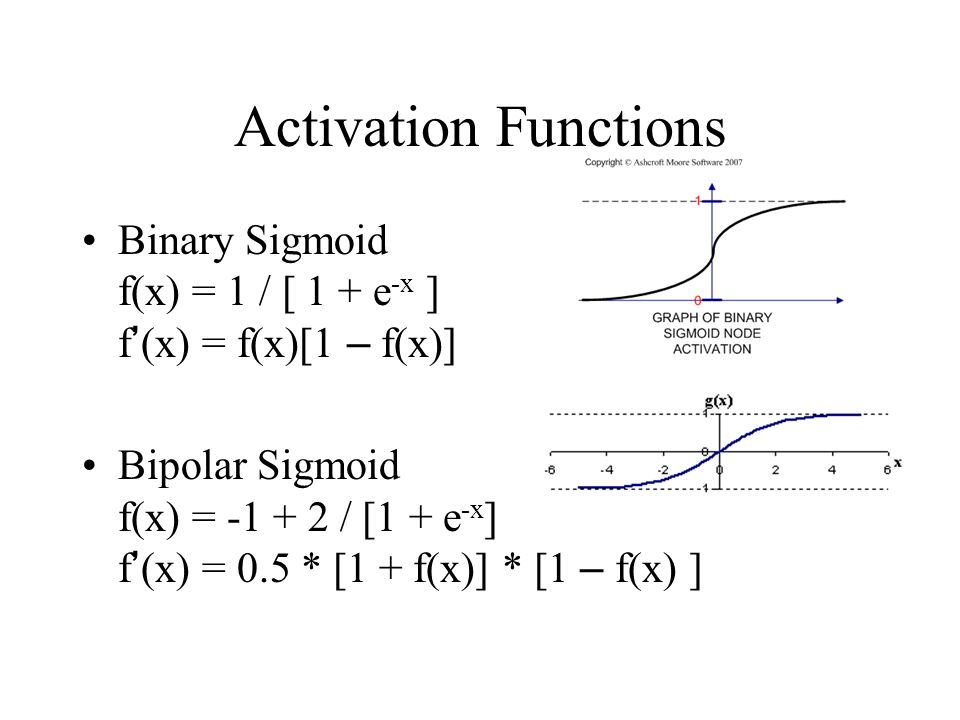Image Source: https://slideplayer.com/slide/10997682/

There were various problems with sigmoid and hyperbolic tan activation functions and that’s why we need to see a few more activation functions such as ReLu, Leaky ReLu, Elu, etc. We are going to describe them below :

(ii) RELU (Rectified Linear Unit): Some problems with sigmoid and Hyperbolic Tan (tanh) activation functions such as Vanishing Gradient Problem and Computational Expensive Problem.

The RELU activation function returns 0 if the input value to the function is less than 0 but for any positive input, the output is the same as the input. It is also continuous but non-differentiable at 0 and at values less than 0 because its derivative is 0 for any negative input.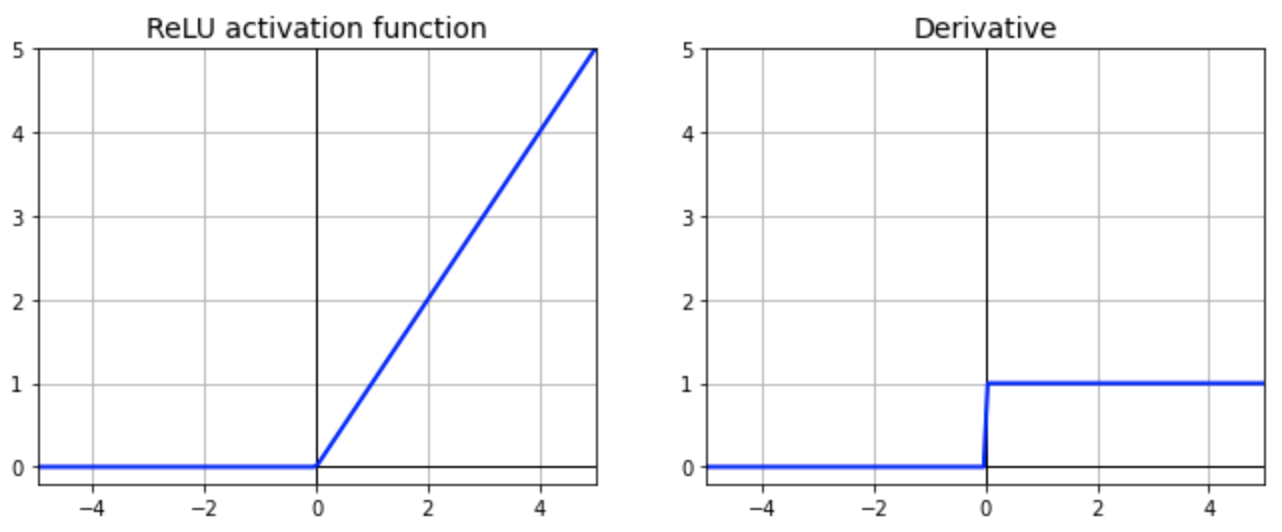Image Source: https://towardsdatascience.com/why-rectified-linear-unit-relu-in-deep-learning-and-the-best-practice-to-use-it-with-tensorflow-e9880933b7ef

Graph:Image Source: Author

Code Snippet:

```import numpy as np
import matplotlib.pyplot as plt
import numpy as np
plt.style.use('seaborn')
plt.figure(figsize=(8,4))
def RectifiedLinearUnit(t):
lst=[]
for i in x:
if i>=0:
lst.append(i)
else:
lst.append(0)
return lst
arr = np.linspace(-5, 5)
plt.plot(arr, RectifiedLinearUnit(arr))
plt.title('Rectified Linear Unit Activation Function')
plt.show()```

Below is a comparison of Tanh and Sigmoid Activation Function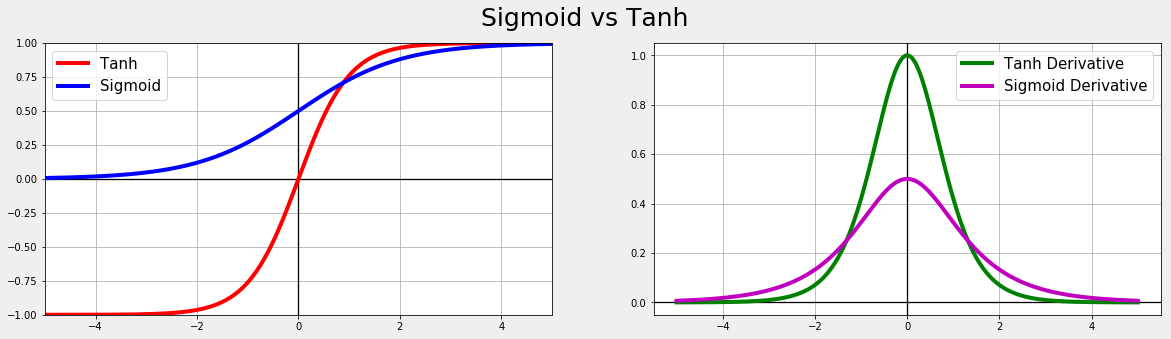Image Source – https://a-i-dan.github.io/math_nn

Sometimes RELU suffers from a problem known as Dying RELU. In some
cases, we may find that half of our Neural Networks Neurons are dead.
This is due to the fact that for many gradients where the input is
negative, the RELU function returns 0 as the output.

To solve this problem, we can use a variant of the RELU function such as LEAKY RELU, Exponential Linear Unit.

(iii) Leaky RELU: in order to solve the DyingRELU problem, people proposed to set the first half of RELU 0.01x instead of 0.

With Leaky RELU there is a small negative slope so instead of that firing at all, for large gradients, our neurons do output some value and that makes our layer much more optimized too.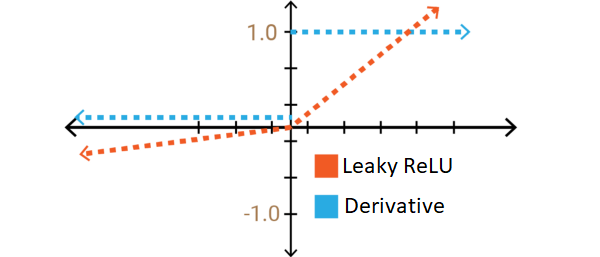Image Source :https://towardsdatascience.com/comparison-of-activation-functions-for-deep-neural-networks-706ac4284c8a

Graph:Code Snippet:

```import numpy as np
import matplotlib.pyplot as plt
import numpy as np
plt.style.use('seaborn')
plt.figure(figsize=(8,4))
def LeakyRectifiedLinearUnit(arr):
lst=[]
for i in arr:
if i>=0:
lst.append(i)
else:
lst.append(0.01*i)
return lst
arr = np.linspace(-5, 5)
plt.plot(arr, LeakyRectifiedLinearUnit(arr))
plt.title('Leaky ReLU Activation Function')
plt.show()```

(iv) ELU (Exponential Linear Unit) function: It is the same as RELU for positive input, but for negative inputs, ELU smoothes slowly whereas RELU smoothes sharply.

Some of the features of ELU activation are:

• The mean of output is close to 0
• One small problem is that it is slightly more computationally intensive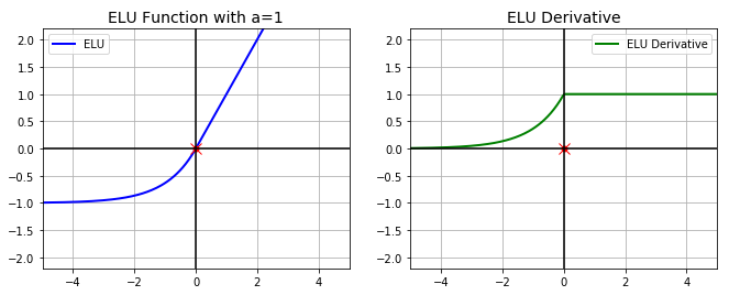Image Source: https://medium.com/@kshitijkhurana3010/activation-functions-in-neural-networks-ed88c56b61

Code Snippet:

```import numpy as np
import matplotlib.pyplot as plt
import numpy as np
plt.style.use('seaborn')
plt.figure(figsize=(8,4))
def elu(arr):
lst=[]
for i in arr:
if i<0:
lst.append(0.5*(np.exp(i) -1))
else:
lst.append(i)
return lst
arr = np.linspace(-5, 5)
plt.plot(arr, elu(arr))
plt.title('ELU Activation Function')
plt.show()```

(v) Softmax Function: it not only maps our output to [0,1] range but also maps each output in such a way that the total sum is 1. The output of SoftMax is therefore a probability distribution.

It is often used in the final layer of a Neural Network for a multiclass classification problem.

For example, let us suppose that the output of the last layer was {40,30,20} of the following Neural Network.

So when we apply the SoftMax function in the output layer: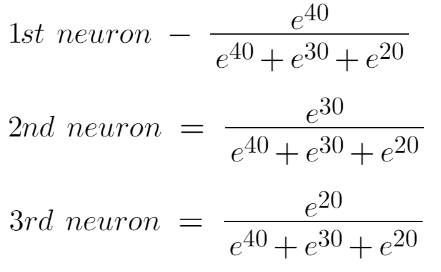Image source: Author

In conclusion, softmax is used for multiclass classification in the logistic regression model whereas sigmoid is used for binary classification in the logistic regression model.

Graph: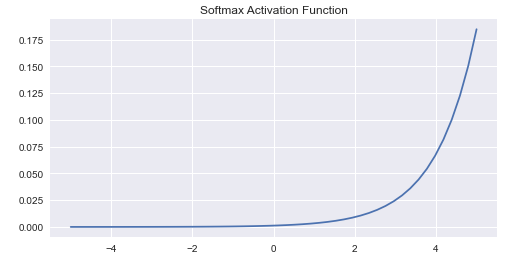Code Snippet:

```import numpy as np
import matplotlib.pyplot as plt
import numpy as np
plt.style.use('seaborn')
plt.figure(figsize=(8,4))
def softmax(t):
return np.exp(t) / np.sum(np.exp(t))
t = np.linspace(-5, 5)
plt.plot(t, softmax(t))
plt.title('Softmax Activation Function')
plt.show()```

## Some Problems in Adding more hidden layers

As more layers using certain activation functions are added to NN, the gradients of the loss function approach zero making the network hard to train.

Certain activation functions like “Sigmoid Function” squish large input space to small input space between 0 and 1. The derivative of the sigmoid function ranges between 0 and 0.25.For shallow networks, this is not a big problem. However, when more layers are used, it can cause the gradient to be too small which makes wnew approximately equal to Wold.

A small gradient means that the weights are biases of the initial layers will not be updated effectively with each training session.

This is why we don’t use the sigmoid activation function. Activation functions like RELU and its variants don’t cause small derivatives.

In a network of n hidden layers, n derivatives will be multiplied together. If the derivatives are large then the gradient will increase exponentially as we propagate down the model until they eventually explode and this problem is called exploding gradient problem.

The exploding gradient can cause problems in the training of ANN. When there is exploding gradient an unstable network can result and the learning cannot be completed. The values of the weights can also become so large as to overflow and result in something called NaN values.

Some solutions to this problem are:

1. Reducing the number of layers

2. Proper weight initialization techniques

## EndNotes

Congrats on completing the second article in this series!

We started by introducing you to what gradient descent is. We have then read about a few other important concepts like various types of activation functions, when to use them, their graphs, and code snippets so that you can implement them yourselves too.

Now that you have your foundations established, in the next article, we will dive deep into Back Propagation to see how exactly it works and what is the math behind it. It is one of the most important topics of Artificial Neural Networks and we couldn’t be more excited to share more with you soon.

This article has been written by 2 authors named Varin Anand and Anshul Saini.

Varin is an undergraduate student currently majoring in Information Technology. (BTech in IT). He is interested in the field of Software Development and Data Science.

Anshul is an undergraduate student currently in his last year majoring in Statistics (Bachelors of Statistics) and have a strong interest in the field of data science, machine learning, and artificial intelligence. We are constantly learning and motivated to try new things.

We are open to collaboration and work.